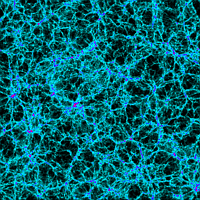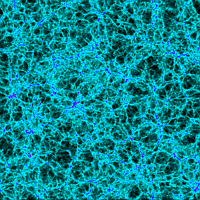We have done
 Very Large Simulations

 Introduction

Two cosmological models were simulated in larger boxes (479 Mpc/h for LCDM and 321 Mpc/h for tCDM) than those of the Virgo simulations, while keeping a high mass resolution. We find many clusters in the box, which are still well resolved and thus suitable for re-simulation. The main projects associated with these simulations are:

 Simulation parameters

Shown in the left panels are projected mass distibutions in a sliced region in the simulation box. Low-density regions which look like "voids" are dark, while blue and red parts are high-density regions. The pictures were created using an SPH smoothing scheme which assigns to each particle an individual smoothing length.Boxsize 479 Mpc/h 5123 particles OMEGA = 0.3 LAMBDA = 0.7 H0 = 70 km/(Mpc sec) Sigma8 = 0.9 Mass per particle 6.86 x 1010M_sun/hBoxsize 320.6 Mpc/h 5123 particles OMEGA = 1.0 LAMBDA = 0.0 H0 = 50 km/(Mpc sec) Sigma8 = 0.51 Mass per particle 6.86 x 1010M_sun/h

 Reference